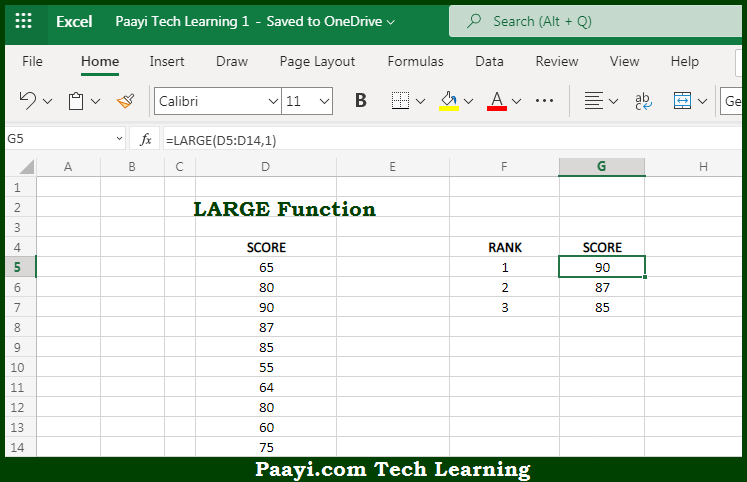# Learn How to Use Microsoft Excel LARGE Function

Written by | 0 Comments | 453 Views

In this article, you will learn how to use the Microsoft Excel LARGE function and its prime function in Microsoft Excel. You will also get to know the Microsoft Excel LARGE function return value and syntax with the help of some examples.

Microsoft Excel LARGE Function

The main purpose of the Microsoft Excel LARGE function is to get the nth largest value. That implies, with the help of the LARGE function you can able to return numeric values based on their position in a list when sorted by value. It can be understood as, it can retrieve "nth largest" values that is - 1st largest value, 2nd largest value, 3rd largest value, etc. So, with the help of the LARGE function, you can able to get the nth largest value in the array.

Return Value of LARGE Function

The return value will be the nth largest value in the array.

Syntax of LARGE Function

=LARGE(array, n)

Where the arguments:

• array: This is the array or range from which you want to get the nth largest value.
• n: This is the integer that specifies the position from the largest value, that is. the nth position.

## How to Use Microsoft Excel LARGE Function?So we know that Microsoft Excel LARGE function you can able to get the nth largest value. That implies, with the help of the LARGE function you can able to return numeric values based on their position in a list when sorted by value. It can be understood as, it can retrieve "nth largest" values that is - 1st largest value, 2nd largest value, 3rd largest value, etc. So, with the help of the LARGE function, you can able to get the nth largest value in the array.